Home > GC > Chapter 8 > Lesson 8.1.4 > Problem8-39

8-39.
1. Find the area of each figure below. Show all work. Homework Help ✎

1.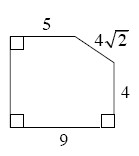2.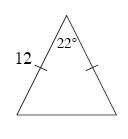3.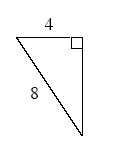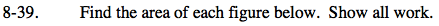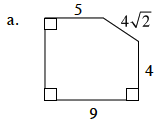$\text{Create a triangle that would make }4\sqrt{2}\text{ the hypotenuse and }$

$4\text{ and }x\text{ each of the triangle's bases.}$

Now use the Pythagorean Theorem to solve for x.

Now that you know the value of x, you can find the area of the triangle and the area of the rectangle that have been created.

Find the area of the figure by subtracting the area of the triangle from the area of the rectangle.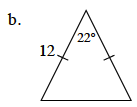Draw a height from the 22° angle, Since the triangle is isosceles, what properties are true about the two created right triangles?

A ≈ 27 units²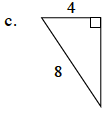Use the Pythagorean Theorem to first find the unknown side and then the triangle's area.